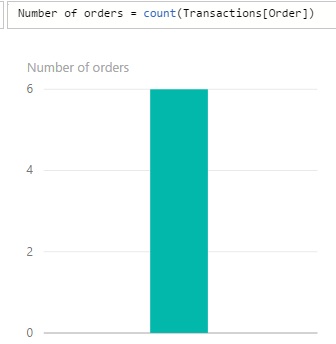# Introduction to DAX (Data Analysis Expressions)

DAX, or Data Analysis Expressions, is a library of functions, formula and query language used in analytics. It is used in:

• Power BI
• SSAS Tabular models (SQL Server Analysis Services)
• Power Pivot in Excel

To write DAX formulas in Power BI, open Power BI Desktop and go to your dataset. In the example below we have Transactions: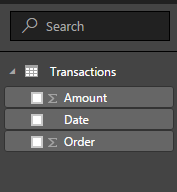Click on the Ellipse next to the dataset and you will see “New measure” and “New column”: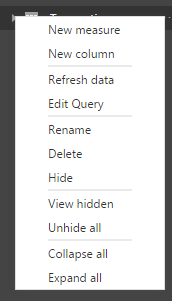When you make a selection, for example New measure, a formula bar will appear: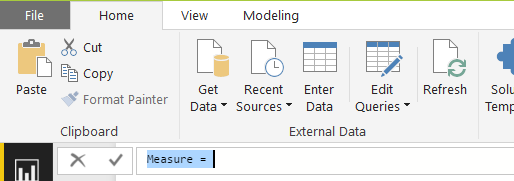You can then rename the measure (the name defaults to Measure) and provide a formula.

A simple example, let’s say we want to know the number of transactions in the dataset. Transactions in this case refers to sales orders. We can give the measure a new name and then start typing in the DAX formula. As we type, we see intellisense helping: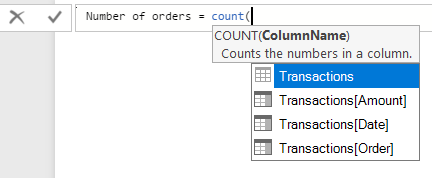Once complete, DAX will tell us if the formula is correct or not. If incorrect, we can fix it. If not, we can use the measure as a visualization or other.